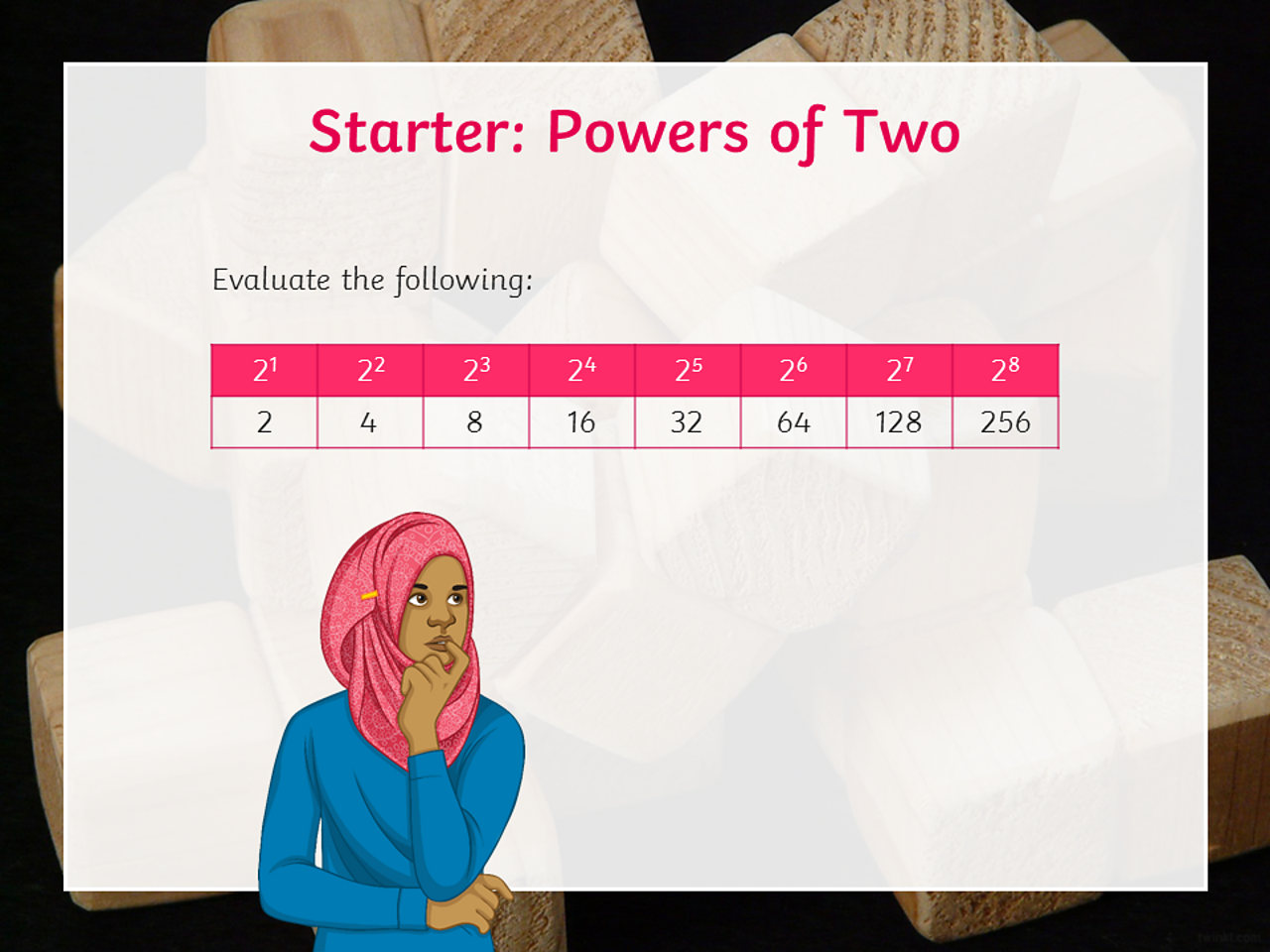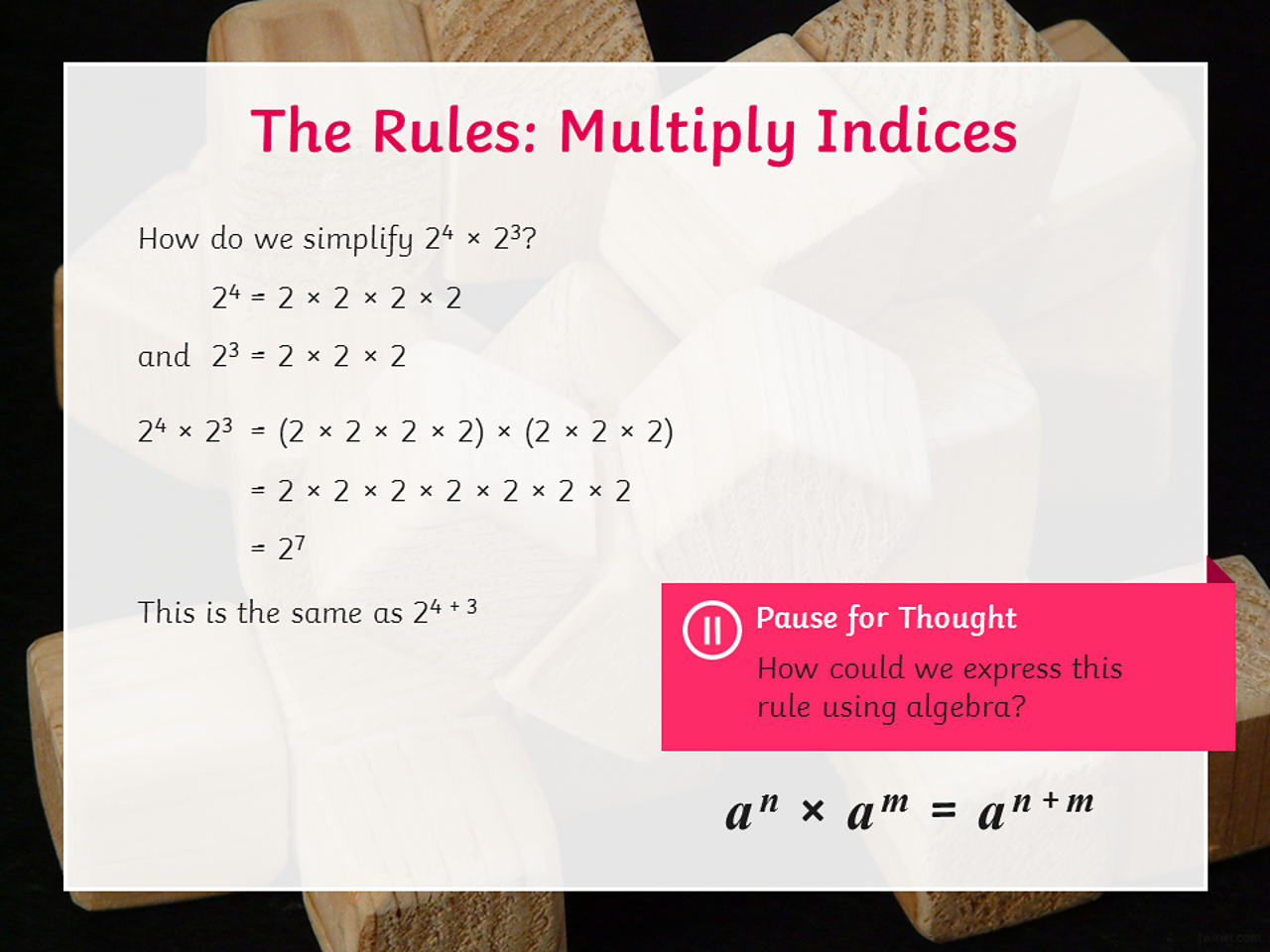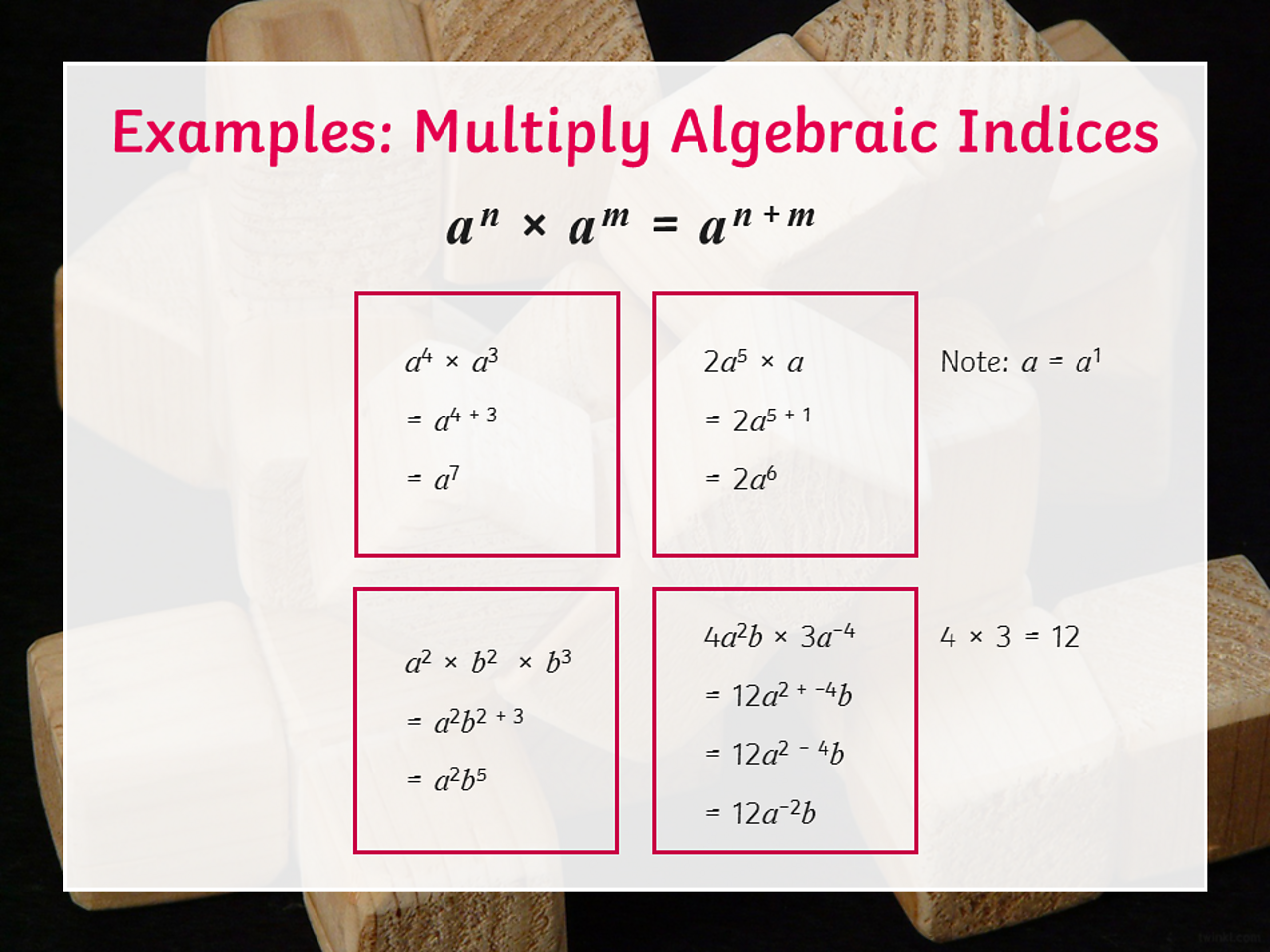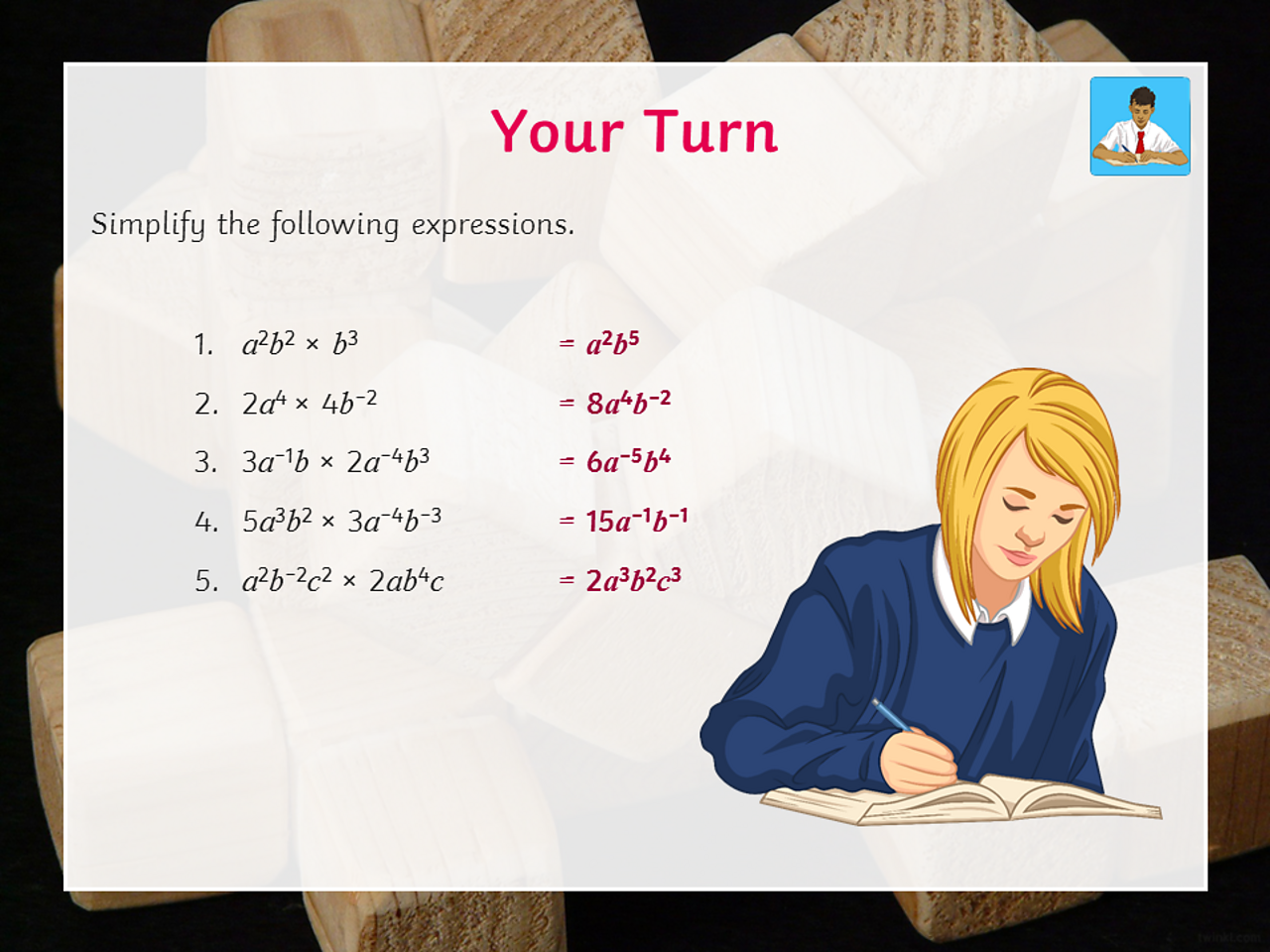# How to simplify algebraic expressions involving multiplication and powers

## Home learning focus

In this lesson, you'll learn how to simplify algebraic expressions involving multiplication and powers.

This lesson includes:

• two slideshows

• two activities

Created in partnership with Twinkl.

# Learn

These slides will show you the conventions to use when multiplying algebraic terms.

In the following slides, you'll learn to simplify algebraic expressions involving indices. Indices are the plural of index. The index of a number tells you how many times to use it in a multiplication.Evaluate the following: 2¹, 2², 2³, 2⁴, 2⁵, 2⁶, 2⁷, 2⁸.1 of 4What is 2⁴ × 2³ ? You can add the powers together, so 4 + 3 = 7, so 2⁴ × 2³ = 2⁷.2 of 43 of 4Here are a few expressions for you to try simplifying.4 of 4

# Practise

## Activity 1

Worksheet - Building pyramids

Practise building pyramids to simplify the expressions using multiplication with this worksheet from Twinkl.